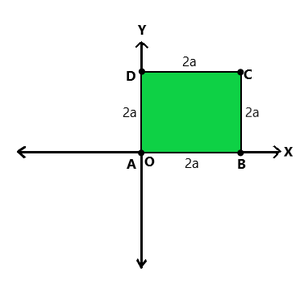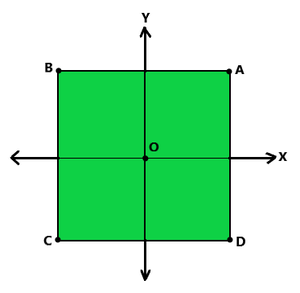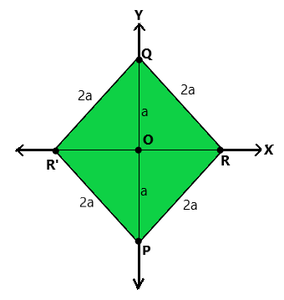GeeksforGeeks App
Open AppBrowser
Continue

## Related Articles

• RD Sharma Class 10 Solutions

# Class 10 RD Sharma Solutions- Chapter 14 Coordinate Geometry – Exercise 14.1

### Problem 1: On which axis do the following points lie?

(i) P (5, 0)

Solution:

As its ordinate is 0. So, it lies on x-axis.

(ii) Q (0, -2)

Solution:

As its abscissa is 0. So, it lies on y-axis (negative half).

(iii) R (-4, 0)

Solution:

As its ordinate is 0. So, it lies on x-axis (negative half).

(iv) S (0, 5)

Solution:

As its abscissa is 0. So, it lies on y-axis.

### (ii) The centre of the square is at the origin and coordinate axes are parallel to the sides AB and AD respectively.

Solution:(I)(II)

(i) Coordinate of the vertices of the square ABCD of side 2a will be – A(0, 0), B(2a, 0), C(2a, 2a) and D(0, 2a)

(ii) Coordinate of the vertices of the square ABCD of side 2a will be – A(a, a), B(-a, a), C(-a, -a) and D(a, -a)

### Problem 3: The base PQ of two equilateral triangles PQR and PQR’ with side 2a lies along y- axis such that the mid-point of PQ is at the origin. Find the coordinates of the vertices R and R’ of the triangles.

Solution:Here, We have two equilateral triangles PQR and PQR’ with side 2a lying along y-axis.

O is the mid-point of PQ.

Now, in ∆QOR, ∠QOR = 90°

Now, By using Pythagoras theorem –

OR2 + OQ2 = QR2

OR2 = (2a)2 – (a)2

OR2 = 3a2

OR = (√3)a

Thus, the coordinate of vertex R is (√3 a, 0) and coordinate of vertex R’ is (-√3 a, 0)

My Personal Notes arrow_drop_up
Related Tutorials